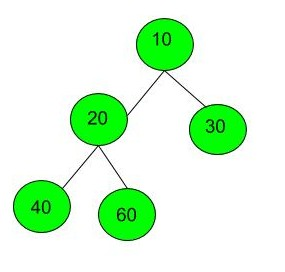Left View of Binary Tree
Easy Accuracy: 37.86% Submissions: 100k+ Points: 2

Given a Binary Tree, print Left view of it. Left view of a Binary Tree is set of nodes visible when tree is visited from Left side. The task is to complete the function leftView(), which accepts root of the tree as argument.

Left view of following tree is 1 2 4 8.

1
/     \
2        3
/     \    /    \
4     5   6    7
\
8

Example 1:

Input:
1
/  \
3    2
Output: 1 3



Example 2:

Input:Output: 10 20 40


You just have to complete the function leftView() that prints the left view. The newline is automatically appended by the driver code.

Expected Time Complexity: O(N).
Expected Auxiliary Space: O(Height of the Tree).

Constraints:
1 <= Number of nodes <= 100
1 <= Data of a node <= 1000